# 1. 基本概念

• 学习分类：基于树的算法被认为是最好的，最常用的监督学习方法之一
• 优势
• 基于树的算法使预测模型具有较高的准确性，稳定性和易解释性
• 与线性模型不同，它们很好地映射了非线性关系
• 用途
• 解决分类问题（classification：categorical variables ）
• 解决回归问题（regression：continuous variables ）
• 缩略语

MSE Mean Squared Error 均方误差
MAE Mean Absolute Error 平均绝对误差
OOB Out Of Bag

## 1.1 集成学习 (Ensemble Learning)

Boosting 各个弱学习器之间有依赖关系
Bagging 各个弱学习器之间没有依赖关系，可以并行拟合

### 1.1.1 Bagging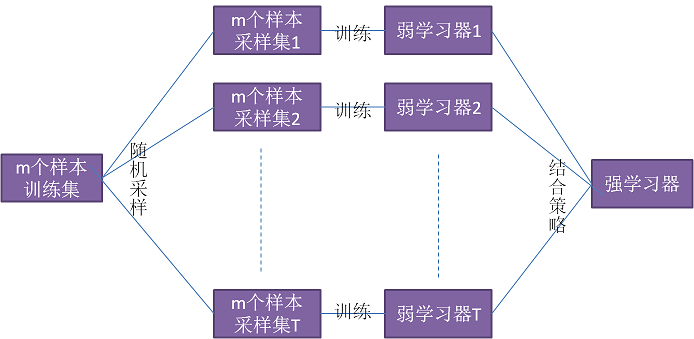### 1.1.2 Boosting

• 提升树(boosting tree)系列算法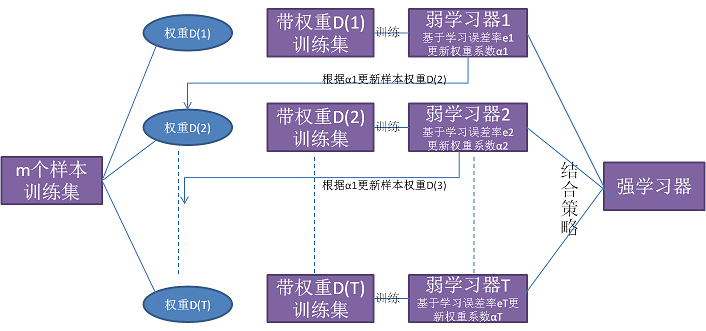# 2. 决策树（Decision Trees）

• 构造决策树的基本思想
• 随着树深度的增加，节点的熵迅速降低
• 熵降低的速度越快越好，这样就可得到一棵高度最矮的决策树
• 寻找最佳划分点
• 划分属性
• 划分属性的取值
• 每一种划分，都可以计算出基于 划分特征= 某个特征值， 将样本集合D划分为两个子集
• 使用三种算法生成不同的决策树

1 ID3 信息增益
(取最大值)
1）取值多的属性，更容易使数据更纯，其信息增益更大
2）训练得到的是一棵庞大且深度浅的树：不合理
3）使用了大量的对数运算，计算量大

2 C4.5 信息增益率
(取最大值)
1) 采用信息增益率替代信息增益
2) 取值少的属性
3) 使用了大量的对数运算，计算量大

3 CART 基尼系数
(取最小值)
1）以基尼系数替代熵
2）最小化不纯度，而不是最大化信息增益
3）每次选择最优属性后，再选择该属性的一个取值进行划分

• 训练阶段
• 从给定的训练数据集D， 构造出一查决策树
$class = DecisionTree(D)$
• 分类或回归阶段
• 从根开始，按照决策树的分类属性逐层往下划分，直到叶节点，获得概念（决策、分类）结果
$y = DecisionTree(x)$

## 2.1 概念

• 决策树：是一种预测模型，该模型使用一组二进制规则来计算目标值
• 使用不同的算法确定节点上的最佳分割
• 优点
• 易于解释决策规则
• 非参数，因此很容易合并一系列数字或分类数据层，并且无需选择单峰训练数据
• 对训练数据中的异常样本具有很好的鲁棒性
• 一旦规则确定，分类速度很快
• 可以处理非线性特征
• 考虑了特征之间的相互作用
• 缺点
• 决策树倾向于过拟合训练数据，泛化能力差
• 垂直于特征空间轴分割并不总是有效的
• 无法预测超出训练数据中响应变量的最小和最大限制

### 2.1.1 信息熵（Entropy）

• 信息熵（entropy）：表示信息不确定性程度，不确定性越大，熵越大

• 概率越大，信息熵越小
• 概率越小，信息熵越大
• 纯度(purity)： 纯度越高，信息熵越小，概率越大

• 单个样本数据集D的熵 (样本被分为K类)
$H(D) = Ent(D) = -\sum_{k=1}^K p_k \cdot \ln{p_k}$

• $p_k$：在样本数据集D中(有K类样本)，第$k$类样本所占的比例
• 多个样本数据集的熵 (有V个样本数据集)
$Ent(D_1, D_2, ..., D_V) = \sum_{v=1}^V \frac {|D_v|}{|D|} Ent(D_v)$

• $D = D_1 \cup D_2 \cup ... \cup D_V$
• $|D_v|$：表示集合$D_v$中的样本个数
• $|D|$：表示集合$D$中的样本个数

### 2.1.2 信息增益

• 物理含义：表示向纯度方向迈出的步长 ，迈得越快越好
• 公式：
$g(D,a)=Gain(D,a) = Ent(D) - \sum_{v=1}^V \frac{|D_v|}{|D|}Ent(D_v)$
• 划分前的信息熵 减去 划分后的信息熵
• $a$：表示属性
• 假定属性$a$$V$种取值，因此输入数据集合D, 根据属性a可以将D划分为V个分支集合
• 那么根据属性$a$划分得到的信息增益为$Gain(D,a)$
• $D_v$：表示根据属性$a$的某个取值$a_v$得到的分支集合
• $Ent(D)$：划分前的信息熵
• $\sum_{v=1}^V \frac {|D_v|}{|D|} Ent(D_v)$：划分后的信息熵
• $\frac {|D_v|}{|D|}$：第$v$个分支的权重，样本越多越重要，即本分支样本个数除以D中的样本总数
• $Ent(D_v)$：第$v$分支样本集合的信息熵
• 应用
• 信息增益越大，则意味着使用属性a进行划分带来的纯度提升越大，因此可以根据信息增益选择属性
• 因为划分后的纯度越高, 那么信息熵越小, 即第二项越小, 因此增益越大

## 2.2 如何构建决策树

• 构造过程
• 决策树的特征选择
• 决策树的生成（ID3，C4.5）
• 决策树的剪枝

### 2.2.1 树长到何时停？

• 当前结点包含的样本全属于同一类别，无需划分
• 当前结点包含的样本集合为空，不能划分

### 2.2.2 树如何长？

#### 2.2.2.1 ID3算法 （根据信息增益划分）

• 长的过程
• 在根节点处计算信息熵
• 然后根据属性依次划分并计算其节点的信息熵，用根节点信息熵–属性节点的信息熵=信息增益
• 根据信息增益进行降序排列，排在前面的就是第一个划分属性
• 其后依次类推，这就得到了决策树的形状，也就是怎么“长”了
• 局限性
• 对可取值数目较多的属性有所偏好 （如对无意义的且唯一的ID编号，其信息增益每次都是最大的）

#### 2.2.2.2 C4.5算法（根据信息增益率划分）

• 为解决信息增益的问题（即ID3的局限性），引入了信息增益率

• 信息增益率
$Gain_ratio (D,a) = \frac{Gain(D,a)}{IV(a)}$
其中$IV(a) = -\sum_{v=1}^V \frac {|D^v|} {|D|} \ln {\frac {|D^v|}{|D|}}$
-$IV(a)$： 特征a的可能取值数目越多(即V越大)，则IV(a)的值通常就越大

• 信息增益率的本质

• 是在信息增益的基础之上乘上一个惩罚参数
• 具有此特征的样本数较多时，惩罚参数较小
• 具有此特征的样本数较少时，惩罚参数较大
• 局限性

• 信息增益率偏向取值较少的特征
• 信息增益率使用方法

• 基于其局限性，并不是直接选择信息增益率最大的特征
• 而是先在候选特征中找出信息增益高于平均水平的特征
• 然后在这些特征中再选择信息增益率最高的特征

#### 2.2.2.3 CART算法（根据基尼系数划分）

• CART: Classification And Regression Trees
• 基于信息增益及信息增益率的不足，引入了基尼指数
• 集合D的基尼值
$Gini(D) = \sum_{k=1}^K \sum_{k' \ne k} p_k p_{k'} = \sum_{k=1}^K p_k (1-p_k) = 1-\sum_{n=1}^K p_k^2$
• 含义：表示在样本集合中一个随机选中的样本被分错的概率
• Gini(D)越小，数据集D的纯度越高
• 对每一种划分，都可以计算出基于 划分特征= 某个特征值 将样本集合D划分为两个子集的纯度
• 特征a的基尼系数
$GiniIndex(D, a) = \frac {|D_1|}{|D|} Gini(D_1) + \frac {|D_2|}{|D|} Gini(D_2)$
• $a$：为特征
• 根据使基尼系数最小的特征进行选择
• 最后根据叶节点中样本的标签数量, 取多数类别作为该叶子节点的类别
• 对于一个具有多个取值（超过2个）的特征，需要计算以每一个取值作为划分点，对样本D划分之后子集的纯度$Gini(D,ai)$，(其中Ai 表示特征A的可能取值)
• 然后从所有的可能划分的$Gini(D,a_i)$中找出Gini系数最小的划分，这个划分的划分点，便是使用特征A对样本集合D进行划分的最佳划分点。到此就可以长成一棵“大树”了。
• 流程（最小化平方误差）
• 先选择一个属性，再选择一个取值将节点集合分为两个子集
• 对每个子集选择一个输出值使得划分后的两个集合与该输出值的的平方差最小
• 然后根据最小平方差, 选择最优属性最优切分点
• 最后得到多个叶节点，每个叶节点包含多个样本，叶节点的输出值取所有样本输出值的均值或使平方差最小的值。

## 2.3 分类树

• 输入：样本数据
• 输出：离散的类别

### 2.3.1 构建分类树

• 使用训练数据创建模型
• 树生成器确定
• 在节点上拆分哪个变量以及拆分的值
• 决定停止（生成叶节点）或再次拆分
• 将叶节点分配给一个类

### 2.3.2 剪枝(Pruning)

• 过拟合很常见，因为单个像素可能是叶节点
• 分类树可以具有数百或数千个节点，需要通过修剪来减少这些节点以简化树
• 限制树大小的目标参数：
• 最小节点数（minimum node size）
• 一个节点上样本的标准偏差最大化（maximum standard deviation of samples at a node）

## 2.4 回归树（Regression Trees）

• 输入：样本数据
• 输出：连续值

### 2.4.1 特点

• 回归计算预测变量和响应变量之间的关系
• 结构与分类树类似
• 叶节点是预测的函数值
• 预测值限于叶节点中的值

## 2.5 决策树评价函数

• 评介函数
$C(T) = \sum_{t \in leaf} N_t \cdot H(t)$
• $N_t$：为样本$t$类所占的比例
• 越小越好，类似损失函数

# 3. 随机森林（Random Forests）

• Bagging思想
• Bagging是bootstrap aggregating
• 就是从总体样本当中随机取一部分样本进行训练，通过多次这样的结果，进行投票获取平均值作为结果输出
• 这就极大可能的避免了不好的样本数据，从而提高准确度
• 因为有些是不好的样本，相当于噪声，模型学入噪声后会使准确度不高

## 3.1 随机森林是什么?

• 用途：解决决策树泛化能力弱的特点
• 随机森林：是一种基于树模型的Bagging的优化版本，一棵树的生成肯定还是不如多棵树，因此就有了随机森林

## 3.2 Bagging的生成方法

• 采样方式
• 放回抽样
• 生成过程
• 从样本集中通过重采样的方式产生n个样本
• 假设样本特征数目为a，对n个样本选择a中的k个特征，用建立决策树的方式获得最佳分割点
• 重复m次，产生m棵决策树
• 多数投票机制来进行预测

## 3.3 随机森林工作流程

• 来源
• 随机森林：是Bagging的扩展变体
• 它在以决策树为基础构建Bagging集成的基础上，进一步在决策树的训练过程中引入了随机特征选择
• 过程
• 随机选择样本（放回抽样, 行抽样）
• 构建决策树（CART树）
• 随机选择特征(列抽样)
• 随机森林投票（平均）

## 3.4 随机森林与Bagging比较

• 随机选择样本和Bagging相同
• 随机选择特征是指在树的构建中，会从样本集的特征集合中随机选择部分特征，然后再从这个子集中选择最优的属性用于划分，这种随机性导致随机森林的偏差会有稍微的增加（相比于单棵不随机树），但是由于随机森林的‘平均’特性，会使得它的方差减小，而且方差的减小补偿了偏差的增大，因此总体而言是更好的模型
• Bagging使用的是‘确定性’决策树，在选择特征划分结点时，要对所有的特征进行考虑
• 随机森林使用的是‘随机性’特征数，只需考虑特征的子集

## 3.2 随机森林如何工作？

• 随机选择样本子集：选择一个不同的训练数据子集（约2/3，有放回）进行训练，以训练每棵决策树
• 随机选择分类器
• ID3
• C4.5
• CART
• SVM
• LOGISTIC
• 随机选择特征子集
• 随机选择的特征子集用于拆分每个节点
• 变量（特征）的使用数量由用户决定
• 较小的子集产生较少的相关性（较低的错误率），但较低的预测能力（较高的错误率） （即过拟合）
• 最佳值范围通常很广
• 测试数据：用于估计误差变量重要性
• 计算结果
• 投票机制：
• 一票否决制
• 少数服从多数
• 加权多数
• 分类问题：通过所有树的投票数进行类分配
• 回归问题：使用回归结果的平均值进行回归
• 随机森林常用参数
• 输入数据（predictor and response）
• 决策树的数量（Number of trees）
• 每次拆分要使用的变量数（特征数）
• 计算误差及变量的重要性信息
• 有无替换样本

## 3.3 优缺点

• 优点

• 无需修剪决策树
• 自动生成准确性和变量的重要性
• 过拟合不是问题
• 对训练数据中的异常值不太敏感
• 易于设置参数
• 在当前的很多数据集上，相对其他算法有着很大的优势，表现良好。
• 它能够处理很高维度（feature很多）的数据，并且不用做特征选择(因为特征子集是随机选择的)
• 在训练完后，它能够给出哪些feature比较重要
• 训练速度快，容易做成并行化方法(训练时树与树之间是相互独立的)
• 在训练过程中，能够检测到feature间的互相影响
• 对于不平衡的数据集来说，它可以平衡误差
• 如果有很大一部分的特征遗失，仍可以维持准确度
• 缺点

• 回归无法预测训练数据范围外的值
• 在回归中，经常无法准确预测极端值–低估了高点，高估了低点

# 4. 随机森林算法实现

## 4.1.1 训练示意图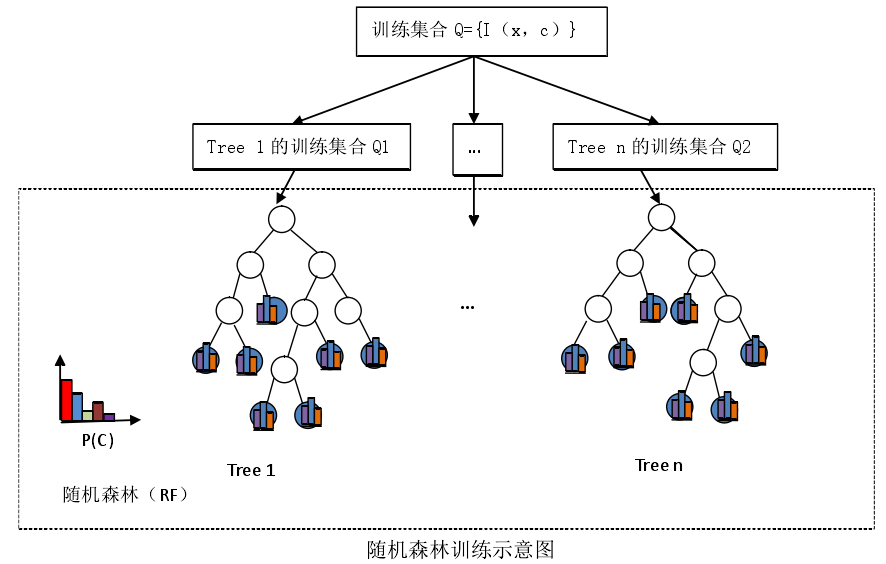## 4.1.2 训练过程

• 给定所有训练图像，把像素灰度值不为0的像素作为训练集合的元素$\{(x, c) \}$
• $(x,c)$中：$x$表示像素, $c$表示类别；$(x,c)$表示像素$x$的类别标签为$c$
• 随机生成参数集合$\{\phi = (u, v, thres) \}$
• $u,v$：表示像素$x$偏移向量
• $thres$：表示非叶子节点中的阈值
• 决策树的训练步骤
• 输入
• 样本集合$Q=\{X,C\}$
• $X=\{x_1, x_2, ..., x_i, ..., x_n \}$：训练像素的集合
• $C=\{ c_1, c_2, ..., c_31\}$：像素所属类别的集合（即人体被细分为31个部位）
• 参数集合$\{(u,v,thres)\}$
• 初始化
• 决策树增长的停止条件包括树的最大深度节点中最小样本数
• 树的增长
• 第一步：判断当前结点是否满足树的停止增长条件，若满足执行步骤二，否则执行步骤三
• 第二步：生成叶子结点 leaf node，计算其中样本集合的类别概率分布 $P(C)$
• 第三步：生成分裂结点，根据参数的度量标准从参数集合中选取最优参数组$(u,v,thres)$，按照（公式 1)把当前节点分裂为左右子节点$Q_{left}$$Q_{right}$
• 第四步：$Q_{left}$$Q_{right}$分别从第一步开始执行
• 输出
• 每个分裂节点中的参数组$(u,v,thres)$
• 所有叶子节点中的概率分布$P(C)$，即类别的统计直方图(类别标签的概率分布)
• 每个叶子节点所表示的是到达该节点所有像素在类别C 上的统计信息
• 即类别分布直方图
• 公式1：根据$(u,v,thres)$判断像素$x_i$的路径
$\begin{cases} x_i \in Q_{left}, & \text{if f_{u,v}(x_i) \le thres} \\[2ex] x_i \in Q_{right}, & \text{if f_{u,v}(x_i) \gt thres} \end{cases}\quad\quad\quad\quad (公式1)$
• 公式2：像素$x_i$的深度差分特征
$f_{u,v}(x_i) = d_I(x_i+ \frac{u}{d_I(x_i)}) - d_I(x_i+\frac{v}{d_I(x_i)}) \quad \quad (公式2)$
• $u和v乘以\frac{1}{d_I(x_i)}$：对偏移量$u,v$进行归一化，以确保与深度无关
• 比如：若u=4, 则在1米处为4个像素，则在2米处则为2个像素，越近相同尺寸的物体像素越多，越远相同尺寸的物体像素越少，为确保与深度无关，深度差应该为始终为人体上相同的两个点的深度差，与距离摄像机的远近无关
• 公式3：选择当前分裂节点的参数组$(u,v,thres)$
• 选择原则：使信息增益（或信息增益率或Gini系数）值最大( 这里使用ID3)
$\phi^* = \argmax_{\phi} InfoGain(\phi) \quad \quad (公式3)$
• $\phi = (u,v,thres)$
• 集合$Q$的熵 ($c_j \in C$)
$Entroy(Q) = - \sum_{j=1}^{|C|} p(c_j)log_{2}\,p(c_j)$
• 信息增益
$InfoGain(\phi) = Entropy(Q) - \sum_{s \in \{ left, right\}} \frac{|Q_s(\phi)|}{|Q_(\phi)|}Entropy(Q_s(\phi))$
• 即通过此$(u,v,thres)$划分之后，两个新样本集合比原样本集合的纯度提高了多少
• 决策树增长过程要解决的关键问题
• 如何从随机生成的参数集合中选取一个参数组，以能够最大程度的来区分当前样本集合，即度量标准 (ID3或C4.5或CART)
• 在保证分类精度、树的结构最简，以及尽量减少训练时间的情况下，确定何时停止树的增长

## 4.2 随机森林分类

• 目的：预测像素的概率分布类别标签

### 4.2.1 分类示意图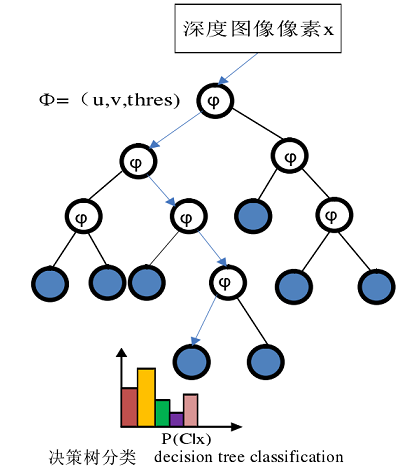### 4.2.2 分类过程

• 设随机森林有 N 棵树，则像素 $x$ 的最终类别概率分布是该像素所到达的 N 个叶子结点中学习了的类别分布的均值，计算公式如下：
$P(C|x) = \frac{1}{N} \sum_{i=1}^N P_i(C|x) \quad\quad\quad (公式4)$
• $P(C|x)$是测试像素的最终类别概率分布，从中选取概率最大值的类别标签$c$ 作为像素 $x$ 的最终类别，即：
$c = \argmax_{c} P(C|x)$
• 伪代码数据结构如下：
• 入口函数：prediction_out = DecisionTree(trained_decision_tree, test_data)
// trained_decision_tree中节点数据结构
typedef struct tree_node_ {
bool split;                              // true: 分裂节点， false: 叶节点
union {
float probability_distribution;  // 类别标签的概率分布
struct {
int u_shift;    // u偏移向量
int v_shift;    // v偏移向量
float thres;       // 分裂阈值
} para;
} data;
} TreeNode;

// test_data数据结构 (包含人体像素的位置和深度信息)
#define MAX_NUM (320*240)
#define POS_X  (0)     // 像素x坐标
#define POS_X  (1)     // 像素y坐标
#define DEPTH  (2)     // 像素深度值
int pixels_data[MAX_NUM];

// prediction_out数据结构（每个人体像素的概率分布）
float prediction_out[MAX_NUM];


## 4.3 随机森林参数分析

### 4.3.1 决策树的深度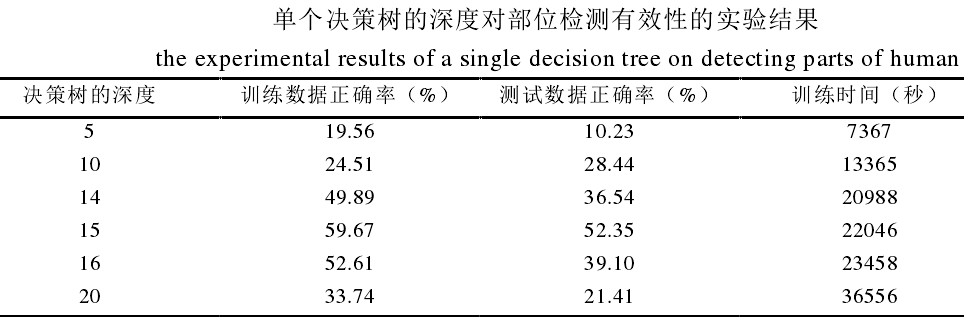### 4.3.2 偏移量对的取值范围

• 据样本统计，每幅图像中人体范围水平方向大约为 50 个像素，垂直方向大约 164 个像素，以 50 为参考值
• 如果选取的范围偏大，则多数像素偏移后会落在人体范围之外，这样极有可能造成树的深度极小，叶子结点个数也极少，从而导致决策树分类的有效性差
• 若选取的范围偏小，极有可能使得相邻的部位检测率低，所以采取了一种折中方案训练决策树时，让随机产生的偏移量对的绝对值范围定在 50 左右
• $u,v \in [-50, 50]$较佳
• 对于单个决策树、树深度为15的条件下的实验结果：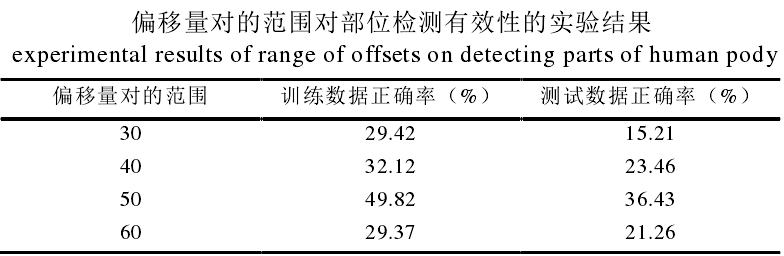### 4.3.3 决策树的个数

• $u,v \in [-50, 50], thres \in [-255,255]$的条件下，决策树的个数分别为1、2、3、4时的部位检测结果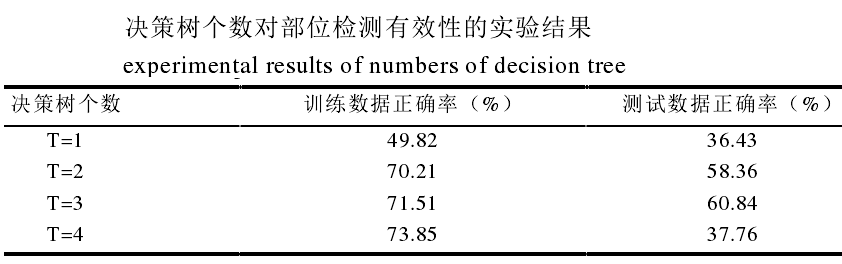• 为什么决策绔树增加时，测试数据正确率反而下降？
• 因为树越多，则随机森林过拟合了
• 尽管随机森林在一定程度上可以避免单个决策树的过拟合，但是当决策树的个数增多时，由于样本被多次选择，容易引起过拟合

### 4.3.4 参数最优值

• 决策树的个数：$2$
• 决策树的深度：$15$
• 偏移向量对的范围：$u,v \in [-50, 50]$
• 阈值范围：$thres \in [-255, 255]$
• 以上参数的实验结果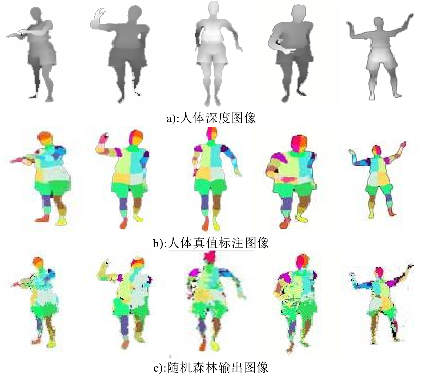# 5. 基于部位检测定位关节位置

• 随机森林：只是学习如何对每个像素进行分类，然后完成测试像素的分类，并不具有定位关节的功能
• 定位关节：通过加权Mean Shift算法实现关节定位

## 5.1 部位合并

• 对于那些细小部位（手、手腕、脚踝、脖子等）而言，由于训练数据中这些较小部位的像素数目仅占很小比例，则该细小部位的正确检测率就会较低
• 属于细小部位的像素容易被划分到相邻主要部位中，比如人的脖子像素容易被划分到相邻的躯干中去，或者相邻的小部位由于其凹凸性不是很明显，所以在同样偏移向量下，提取的深度差分特征值相近，这样极易被错分，比如人的头部的四个部分
• 为了提高对较小部位相应关节的预测精度，在随机森林输出基于 31 个部位的人体标注图像后，把那些占人体像素比例较小的部位划分到相邻的主要部位中（手腕合并到相邻的手部，脖子划分到相邻的躯干、脚踝划分到脚部）
• 相邻的一些部位合并为人体的主要部位（包括左右上臂、下臂臂肘合并为左右臂，左右上躯干合并为人体躯干，左右下躯干合并为人体胯部，左右小腿及脚合并为脚）

## 5.2 算法实现

### 5.2.1 像素分类

• 输入：人体深度图像$I=\{x_1, x_2, ..., x_i, ..., x_n \}$
• 处理：
• 随机森林进行像素分类
• 部位合并
• 输出：得到人体像素分类后的图像
• $I=\{(x_1, c_1), (x_2, c_2), ..., (x_i, c_i), ..., (x_n, c_m) \}, \quad c_i \in C (i=1,2,..., m)$
• 像素$x_i$包含以下信息：
• 像素的类别标签
• 像素在图像中的位置
• 像素的深度值
• 像素所属类别标签的概率
• 在实际场景中，人体部位的各个点(人体部位的凹凸性)在视角范围内，并不在同一个平面 ，所以在三维空间中提取人体关节点更接近真实的人体骨架

### 5.2.2 Mean-Shift(均值偏移)定位关节点

• 用途：寻找概率密度极大的点(局部极大值), 是一种基于非参核密度梯度估计模式匹配算法。
• 基本特征：
• 引入核函数，使得距均值点不同的样本点对其贡献不同
• 赋予样本点一个权重系数，使得不同的样本点重要性不一样
• Mean-Shift 方法仅仅依靠特征空间中的样本点进行分析和处理，算法原理简单，易于并行和实现，由于不需要任何先验知识和进行统计参数估计，使用范围极其广泛
• 概率密度估计沿密度梯度方向递增，直至收敛，最终收敛点即为局部的概率密度极大值点， 可对应特征空间中的一个模式
• 工作原理： Mean shift 算法通过反复迭代搜索特征空间中样本点最密集的区域，最后沿着样本点核密度增加的方向收敛到局部概率密度极大值点

#### 5.2.2.1 核函数

• 假设 X 表示一个 D 维的欧式空间, $\{ x_i \}_{i=1}^n$是该空间中的样本点集合（共有n个样本），每个样本点用列向量$x$表示，则样本点的模为$\Vert x \Vert^2 = x^Tx$
• 若一个函数$K: X \rightarrow R$存在一个剖面(profile) $k:[0, \propto] \rightarrow R$满足以下条件，则称$K(x)$为核函数，其中 R 表示实数域。
$K(x) = k(\Vert x \Vert^2) \quad \quad (公式5)$
• $k$满足：
• 非负的
• 非递增的，即若 $a，则 $k(a) \ge k(b)$
• 分段连续的，并且$\int_0^{\propto} k(r)dr < \propto$

#### 5.2.2.2 核密度

• 假设 X 表示一个 D 维的欧式空间, $\{ x_i \}_{i=1}^n$是该空间中的样本点集合（共有n个样本），$\mathbf x$表示空间中的一个点， $K_{H}(x)$表示核函数，则点$\mathbf x$的密度估计为：
$\hat f(x) = \frac{1}{n} \sum_{i=1}^n K_H(x - x_i) \quad \quad (公式6)$

• 其中$K_H(x) =|H|^{-\frac{1}{2}} K [H^{-\frac{1}{2}} (x)]$
• $H$：表示一个$d \times d$的正定对称矩阵，为了简化计算，一般将其表示为正比于单位阵的矩阵，即$H=h^2I$，使用该 H 是为了增加密度估计的灵活性，则$\hat f(x)$可表示为：
$\hat f(x) =\frac{1}{nh^d} \sum_{i=1}^n K(\frac{x - x_i}{h}) \quad \quad (公式7)$
• $K(x)=c_{k,d}k(\Vert x^2 \Vert)$，其中系数$c_{k,d}$是为了保证核函数在空间中的积分为 1，则$\hat f(x)$变为:
$\hat f(x) = \frac{c_{k,d}}{nh^d} \sum_{i=1}^n k(\Vert \frac{x-x_i}{h} \Vert^2) \quad \quad (公式8)$
• 对上式中的$h$求导，可得：
$\nabla f_{h,K} (x) = \frac {2c_{k,d}}{nh^{d+2}} \sum_{i=1}^n k' (\Vert \frac{x-x_i}{h} \Vert^2) (x-x_i) \quad \quad (公式9)$
• $g(x) = -k'(x)$，结合公式1知g(x)的核函数为$G(x) = c_{k,d}g(\Vert x^2 \Vert)$将其代入公式，得：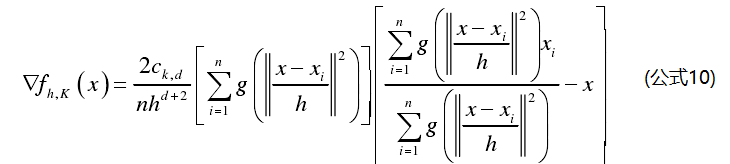• 其中把第一个大括号中的项定义 为点$x$的Mean Shift向量，即：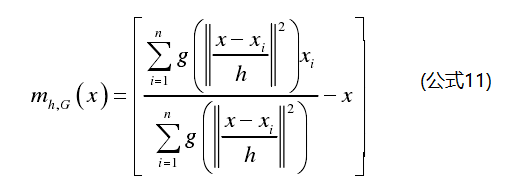• 在点$x$处的基于$G(x)$函数人概率密度估计为：
$f_{h,G} = \frac{c_{k,d}}{nh^d}[\sum_{i=1}^ng(\Vert \frac{x-x_i}{h} \Vert^2)] \quad (公式12)$

• 把公式 11和公式12代入到公式 10 并且进行移位变换后，发现$m_{h,G}(x)$ 与概率密度估计 $\nabla_{h,K}(x)$的方向就一致指向密度增加最大的方向

• $g(x)=1$时，则变为Mean Shift的基本形式，即点$x$的均值偏移量：
$m(x) = \frac{1}{n}\sum_{i=1}^n (x - x_i)$• 上图中的实心点表示点$x$，空心点表示采样点$x_i$，虚线箭头表示采样点与基准点间相对偏移量，即： $x_i-x$ ，这些偏移量的平均值用实线箭头表示，即均值偏移量。

• Mean Shift算法的基本思想：数据点在均值偏移量方向上的重复移动过程，直到收敛过程极值点

• 核函数$G(x)$的用途：在一般情况下，由于样本点距基准点的距离不同，则对均值偏移量的贡献也不相同，所以Cheng 在基本 Mean Shift 算法中引入了核函数$G(x)$的概念

• 权重系数$g$的用途：考虑到每个样本点对均值偏移量的不同程度的影响，因此，为每个样本点还赋予了一个权重$w(x_i)$ ，如公式11中的$g(\Vert \frac{x-x_i}{h} \Vert^2)$就是样本点$x_i$的权重系数

• 总结：

• 给定样本空间、核函数$G(x)$、初始点$x$ 及搜索半径 $h$ 、停止搜索条件$\varepsilon$ ，则基于核函数及权重系数的 Mean Shift 算法的一般步骤：
• 1）使用公式11计算$m_{h,G}(x)$
• 2）若$\Vert m_{h,G}(x) \Vert \gt \varepsilon$, 把$x+m_{h,G}(x)$赋给$x$，继续执行 第1）步，否则停止搜索，输出 $x$

# 参考

11-251万+

#### 机器学习之决策树（Decision Tree）&随机森林（Random forest）04-16150

#### 决策树与随机森林Decision Tree Random Forest

05-2514万+

#### 随机森林（原理/样例实现/参数调优）

12-121018

#### 基于决策树（Decision Tree）的bagging算法：随机森林（Random Forest）（包括具体代码）

08-302256

#### 决策树Decision Tree 和随机森林RandomForest基本概念（一）

11-183539

#### 决策树和随机森林用 python treeinterpreter实现

08-212206

#### 决策树（Decision Tree）

12-133362

#### 机器学习： 决策树（Decision Tree）

04-022万+

#### 基于Unity行为树设计与实现的尝试©️2020 CSDN 皮肤主题: 大白 设计师: CSDN官方博客点击重新获取扫码支付1.余额是钱包充值的虚拟货币，按照1:1的比例进行支付金额的抵扣。
2.余额无法直接购买下载，可以购买VIP、C币套餐、付费专栏及课程。余额充值